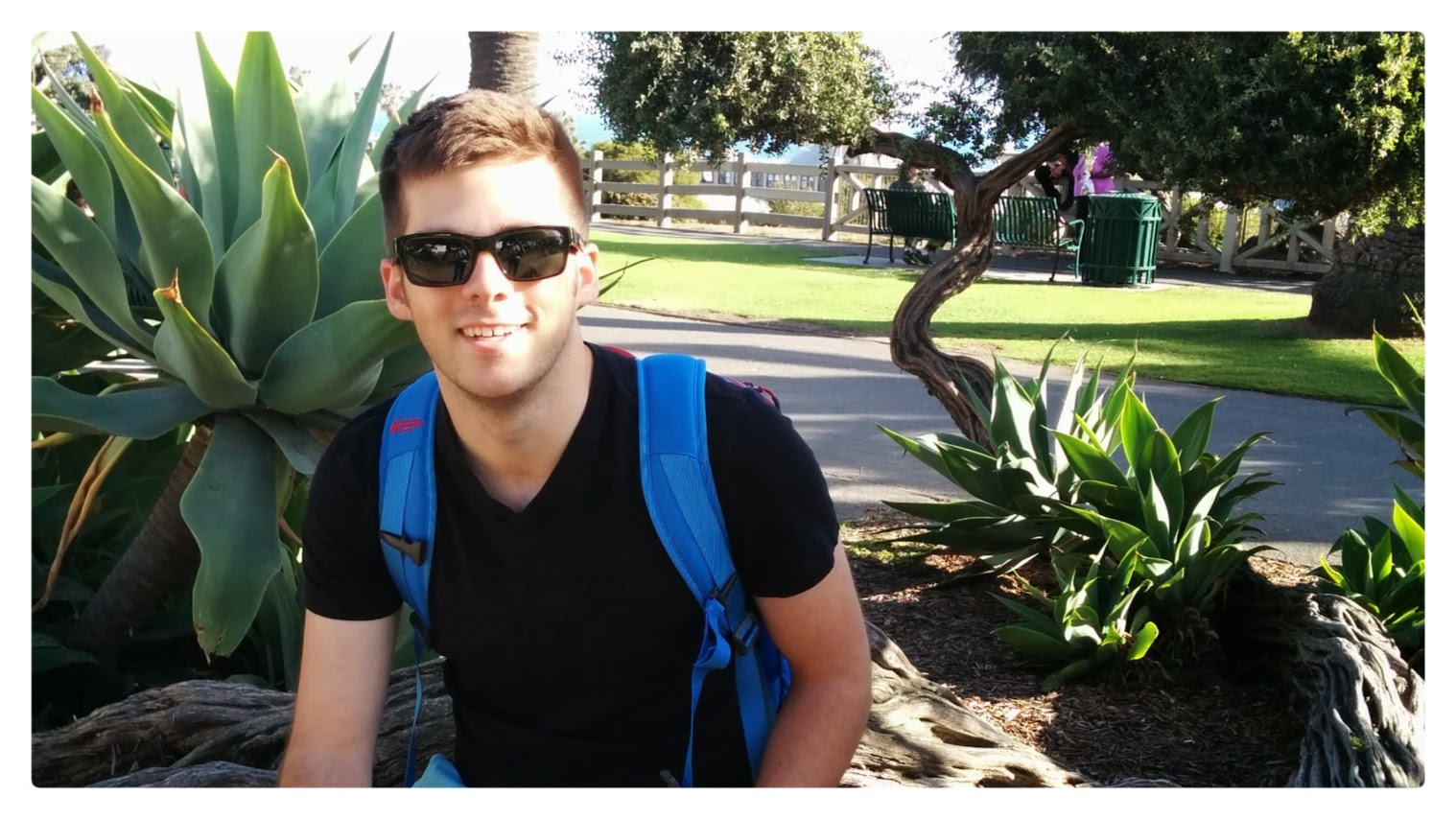I am an assistant professor at the University of Maine. Previously, I was limited-term faculty at the University of Georgia working with the discrete analysis group, and before that I was a Chowla Research Fellow at Penn State, working with Bob Vaughan. Before that, I was a PhD student supervised by John Friedlander at the University of Toronto. View my CV. My primary interests are number theory, combinatorics and harmonic analysis, but I like a good problem from any branch of math. Outside of math I like baseball, snowboarding, golf, playing the guitar and video games. I am a fan of the Ottawa Senators and the Toronto Blue Jays.

Research

I am interested in questions lying somewhere in the realm of analytic number theory, additive combinatorics and harmonic analysis. I have a particular fondness for questions about equidistribution, as well as (additive combinatorial) inverse problems. Below you can find my preprints and publications.
1. (With O. Roche-Newton and S. Senger) Convexity, Superquadratic Growth, and Dot Products. Submitted. arXiv
2. (With P. Bradshaw and M. Rudnev) Higher convexity and iterated second moment estimates. Submitted. arXiv
3. (With G. Petridis) A question of Bukh on sums of dilates. Discrete Analysis, 2021. arXiv
4. (With O. Roche-Newton and M. Rudnev) Higher convexity and iterated sum sets. Combinatorica, 2021. arXiv
5. Littlewood’s problem for sets with multidimensional structure. IMRN, 2020. arXiv
6. (With G. Petridis) Refined Estimates Concerning Sumsets Contained in the Roots of Unity. Proceedings of the London Mathemathical Society, 2020. arXiv
7. Long regularly-spaced and convex sequences in dense sets of integers. J. Combin. Theory. Ser. A, 2020. arXiv
8. (With O. Roche-Newton and D. Zhelezov) On iterated product sets with shifts II. Algebra and Number Theory, 2020. arXiv
9. (With O. Roche-Newton and D. Zhelezov) On iterated product sets with shifts. Mathematika, 2019.
10. (With R. C. Vaughan) Density of binary quadratic forms. Acta Artihmetica, 2020.
11. Additive Correlation and the Inverse Problem for the Large Sieve. Math. Proc. Camb. Phil. Soc., 2018. arXiv
12. (With A. Zaman) The density of numbers represented by diagonal forms of large degree. Mathematika, 2018. arXiv
13. The Additive Structure of Cartesian Products Spanning Few Distinct Distances. Combinatorica, 2017. arXiv
14. (With R. C. Vaughan and R. Zhang) The Least Number with Prescribed Legendre Symbols. Journal of Number Theory, 2017. arXiv
15. Estimates for Character Sums with Various Convolutions. Acta Arithmetica, 2017. arXiv
16. (With B. Lund and O. Roche-Newton) On distinct perpendicular bisectors and pinned distances in finite fields. Finite Fields and Applications, 2016. arXiv
17. Character sums over Bohr sets. Canadian Math Bulletin, 2015. arXiv
18. Capturing forms in dense subsets of finite fields. Acta Arithmetica, 2013. arXiv
19. (With D. Panario and D. Thompson) Swan-like results for binomials and trinomials over finite fields of odd characteristic. Designs, Codes and Cryptography, 2011.

Teaching

I am currently teaching Math 261, Introduction to Abstract Mathematics. In previous semesters at UGA I taught Math 1113 (Precalculus) and Math 2250 (Calculus 1). At Penn State I taught Math 312 (Real Analysis), Math 110 (Techniques of Calculus), Math 141 (Calculus II) and Math 231 (Vector Calculus I).
In the summer of 2020, I gave a graduate lecture series on Fourier Analysis and Number Theory, with accompanying notes. These notes take from various references including Katznelson's An Introduction to Harmonic Analysis, Helson's Harmonic Analysis and Montgomery's Ten Lectures..., and Tao and Vu's Addtive Combinatorics. These notes may have errors.

Contact

1. Mail:
2. Brandon Hanson
3. Department of Mathematics, University Maine
4. 3334 Neville Hall
5. Orono, ME
6. 04469
1. e-mail:
2. b r a n d o n . h a n s o n 1 "at" m a i n e "dot" e d u